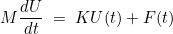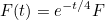## Seldon

Seldon 2016 Seldon 2017

Additional resources with Seldon: HTML Documentation Matrices used for unit tests (folder test/matrix)

## Montjoie

A reduced release of Montjoie can be downloaded here (with only Linear Algebra)

Montjoie Algebra 2017

## Time schemes

Here, you can download a basic implementation in Python of implicit schemes that can be used to solve linear Ordinary Differential Equations (ODEs)

linear_scheme.py

A linear ode (with dense matrices) is considered in a basic example (class ExampleOde) :where M and K are dense matrices, with the arbitrary choiceF is a given vector (constant). Padé schemes can be used to solve this linear ode by typing in Python (once linear_scheme.py and quadrature.py have been downloaded) :

```from numpy import *
from linear_scheme import *

# matrices and source vector
M = rand(5, 5);
K = 0.1*rand(5, 5);
F = rand(5)

# initial condition
Y0 = rand(5)

# ode to solve
ex_ode = ExampleOde(M, K, F)

# if you want to use a Pade scheme, you give the order you want
# type help(PadeScheme) for a detailed help
# here fourth-order Pade scheme will be construct

# SolveOde returns the final solution at time t=2
# of the linear ode M dy/dt = K y(t) + F(t)
# M, K and F are detailed in ex_ode
# 20 is the number of iterations
# syntax : SolveOde(t0, tf, N, Y0, scheme, ode)
# type help(SolveOde) for a detailed help
Yf = SolveOde(0.0, 2.0, 20, Y0, ex_scheme, ex_ode);

# you can also use LinearSdirk
# type help(LinearSdirkScheme) for a detailed help
# the first argument is the order, the second argument the number of extra-stages
ex_scheme = LinearSdirkScheme(4, 1)

# and solve the ode with LinearSdirk
Yf = SolveOde(0.0, 2.0, 20, Y0, ex_scheme, ex_ode);

# a last class has been implemented: LinearRkScheme
# these schemes are explicit Runge-Kutta with optimized coefficients
# to improve the CFL for wave equation discretized with HDG (Hybridizable Discontinuous Galerkin)
# the first argument is the order, the second argument the number of extra-stages
ex_scheme = LinearRkScheme(4, 1)

# and solve the ode with an explicit scheme
Yf = SolveOde(0.0, 2.0, 20, Y0, ex_scheme, ex_ode);

```